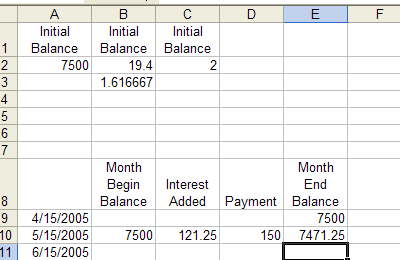### Credit Card Calculator

 In this assignment you'll use Excel to do a simulation of how a credit card can work. This will be a near "worst-case" scenario. You will learn how high interest rates and low monthly minimum payments can lead many people into a trap. (1) Begin a new Excel spreadsheet. (2) Type the following data into the indicated cells.

 Cell What to type Why you typed it. A1 Initial Balance It's just a column heading B1 Interest Rate (%) " C1 Minimum Payment (%) " A2 7500 Initial credit card balance of \$7500 B2 19.4 Yearly interest rate of 19.4% C2 2 Minimum monthly payment of 2% of balance. B3 =B2/12 Divided yearly interest rate into monthly interest rate.

 (3) In cells A9 and A10 we're going to type our first two billing dates. The first billing date will be the 15th of next month and the second will be the 15th of the month after that. Select cells A9 and A10 and fill down to row 500 or so. (4) Then type these headings

 Cell What to type Why you typed it. B8 Month begin balance It's just a column heading C8 Interest added " D8 Payment Due " E8 Month end balance "

(5) Type these formulas

 Cell What to type Why you typed it. E9 =A2 Copies the value from cell A2 into cell E9 B10 =E9 Copies the value from cell E9 into cell B10 C10 =B10*(\$B\$3%) Calculates amount of interest to add D10 =B10*(\$C\$2%) Calculates minimum monthly payment due. E10 =B10+C10-D10 Calculates monthly ending balance.
 At this point this is what you should see:All of the numbers in cells B10, E10, D10 and E10 are the results of the formulas you typed in step 5. (6) Fill cells B10 to E10 all the way down the column. Format all cells as currency as appropriate. Make sure dates in column A are formatted to show the year. (7) Clean up and make it look pretty. Use colors to make sheet easier to read. Make sure all columns are appropriate widths. (8) Save and TURN IN.